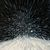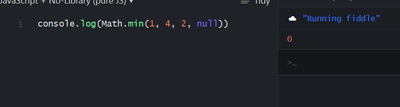# Arcade: Don't treat null as zero in mathematical functions

411
5
02-23-2022 11:53 PM
Status: OpenbyMVP Regular Contributor

Mathematical Functions | ArcGIS Arcade | ArcGIS Developer

While answering a question, I realized that Arcade treats null values as zero in the mathematical functions:

``````var data = [1, 2, 3]
var data_z = [1, 2, 3, 0]
var data_n = [1, 2, 3, null]
function print(f, d, z, n) {
Console("\n" + f)
Console("data: " + d)
Console("data_z: " + z)
Console("data_n: " + n)
}

print("Average", Average(data), Average(data_z), Average(data_n))
print("StDev", StDev(data), StDev(data_z), StDev(data_n))
print("Variance", Variance(data), Variance(data_z), Variance(data_n))
print("Min", Min(data), Min(data_z), Min(data_n))

Console("\nAbs(null): " + Abs(null))``````
```Average
data: 2
data_z: 1.5
data_n: 1.5

StDev
data: 0.816496580927726
data_z: 1.118033988749895
data_n: 1.118033988749895

Variance
data: 0.6666666666666666
data_z: 1.25
data_n: 1.25

Min
data: 1
data_z: 0
data_n: 0Abs(null): 0```

In my opinion, this is wrong. Null means something very different from zero:

• "The temperature today was 0°C" vs. "The thermometer broke, we couldn't measure"
• "I counted 0 cars driving through that street in the last hour" vs. "I was on my lunch break"
• In general: "I know the value, it is zero" vs. "I don't know the value"

I think, null values should not be treated as zero. Instead,

• array functions (like Average, Min, etc.) should ignore them, so that Min([1, 2, 3]) == Min([1, 2, 3, null])
• single-value functions should return null, so that Abs(null) == null
Tags (1)Remove null from the variable?byThe docs even say things like this, for Abs:

Returns the absolute value of a number. If the input is null, then it returns 0.

I wonder what the reason is?

Curiously, I don't see Min behaving that way when using a FeatureSet:

``````var fs = FeatureSet(Text(
{
fields: [{name: 'ints', type: 'esriFieldTypeInteger'}],
geometryType: '',
features: [{attributes: {ints: Null}}, {attributes: {ints: 3}}]
}
))

Min(fs, 'ints')``````

Returns 3.byCuriously, I don't see Min behaving that way when using a FeatureSet

I'm not sure how to feel about that. On the one hand, it shows that it is absolutely possible to ignore the nulls. On the other hand, I'd much rather have the same (albeit wrong) result with both methods.

It gets worse: Calling Abs(\$feature.NullValue) still returns 0, so even that isn't consistent.

``````var fs = FeatureSet(Text(
{
fields: [{name: 'ints', type: 'esriFieldTypeInteger'}],
geometryType: '',
features: [{attributes: {ints: Null}}, {attributes: {ints: 1}}, {attributes: {ints: 2}}, {attributes: {ints: 3}}]
}
))
var values = []
for(var f in fs) { Push(values, f.ints) }

Console("Min")
Console("fs: " + Min(fs, "ints"))
Console("array: " + Min(values))

Console("\nAverage")
Console("fs: " + Average(fs, "ints"))
Console("array: " + Average(values))

Console("\nAbs")
var fs_abs = []
for(var f in fs) { Push(fs_abs, Abs(f.ints)) }
var array_abs = Map(values, Abs)
Console("fs: " + fs_abs)
Console("array: " + array_abs)``````

```Min
fs: 1
array: 0

Average
fs: 2
array: 1.5

Abs
fs: [0,1,2,3]
array: [0,1,2,3]```by

I wonder if it’s consistent with JavaScript, or if Esri diverged and did it their own way?byAnd with averages, there's no built-in JS function to do that, but I'd guess that Esri's is just doing some kind of (sum of array items) / (count of array items) in the background.